Courses

# Describing Motion and Related Concepts Class 9 Notes | EduRev

## Class 9 : Describing Motion and Related Concepts Class 9 Notes | EduRev

The document Describing Motion and Related Concepts Class 9 Notes | EduRev is a part of the Class 9 Course Science Class 9.
All you need of Class 9 at this link: Class 9

MOTION
If we look around us, we find that there are number of objects which are in motion. An object is said to be in motion if it changes its position with the passage of time. In other words, the movement of an object is known as the motion of the object.
Now observe the following bodies or objects and we will be able to understand the meaning of the term "motion". Cars, cycles, motorcycles, scooters, buses, rickshaws, trucks etc. running on the road, Birds flying in the sky, Fish swimming in water. All these objects are in motion. Very small objects like atoms and molecules and very large objects like planets, stars and galaxies are in motion.
Thus, all objects ranging from the smallest atom to the largest galaxy are in continuous motion.

Types of Motion:
(A) Linear motion:  A body has linear motion if it moves in a straight line or path.
Ex.    (i) Motion of a moving car on a straight road.
(ii) Motion of a ball dropped from the roof of a building.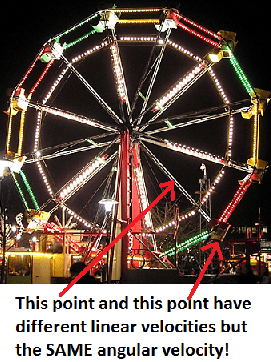Fig: rotating Ferris wheel and different motions

(B) Circular (or rotational) Motion: A body has circular motion if it moves around a fixed point.
A vertical passing through the fixed point around which the body moves is known as axis of rotation.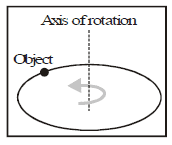Ex.    (i) Motion of an electric fan.
(ii) Motion of merry-go-round
(iii) Motion of a spinning top.

(C) Vibratory motion:
A body has vibratory motion if it moves to and fro about a fixed point.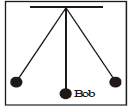Ex.    (i) Motion of a pendulum of a wall clock.
(ii) Motion of a simple pendulum.

MOTION IN LIVING AND NON-LIVING OBJECTS:
It is a common observation that all living objects, whether plants or animals can move in some way or the other.  The motion in animals is more apparent than the motion in plants.
The motion in animals is called LOCOMOTION.
Plants also move but their motion is not apparent as they cannot move from place to place. Their motion takes place in parts. As a plant grows so does its roots and its leaves.

MECHANICS: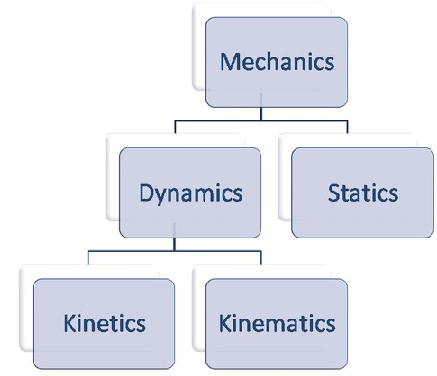Fig: mechanics and its branches The branch of physics which deals with the motion of non-living objects in everyday life is called mechanics or Classical mechanics. It is of two types:-
(i) Statics and (ii) Dynamics

STATICS: Statics deals with bodies at rest under the effect of different forces.
DYNAMICS: Dynamics deals with the bodies in motion. It is further of two types :
(i) Kinematics: Kinematics, which is derived from a Greek word kinema meaning motion,is a branch of Physics, which deals with the motion of a body without taking into account the cause of motion.
(ii) Dynamics proper: Dynamics proper, which is derived from a Greek word dyna meaning power it is a branch of Physics, which deals with the motion of bodies by taking into account the cause of motion (force).

DISTANCE AND DISPLACEMENT
Distance: The length of the actual path between the initial and the final position of a moving object in the given time interval is known as the distance travelled by the object.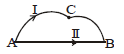Distance = Length of path I (ACB)
Distance is a scalar quantity.

Units-

In SI system: metre (m)
In CGS system: centimetre (cm)
Large unit Kilometre (km)

Displacement:–The shortest distance between the initial position and the final position of a moving object in the given interval of time from initial to the final position of the object is known as the displacement of the object.
Displacement of an object may also be defined as the change in position of the object in a particular direction. That is,

Displacement of an object = Final position – Initial position of the object.
Displacement of an object may be zero but the distance travelled by the object in never zero.
Distance travelled by an object is either equal or greater than the magnitude of displacement of the object.
Displacement = Length of path II (AB) A to B,
displacement is vector quantities.
Units In SI system: metre (m)
In CGS system : centimetre (cm)

Ex. A train goes from station A to station B as shown in figure. Calculate
(i)    the distance travelled by train and
(ii)    the magnitude of the displacement of the train on reaching station B.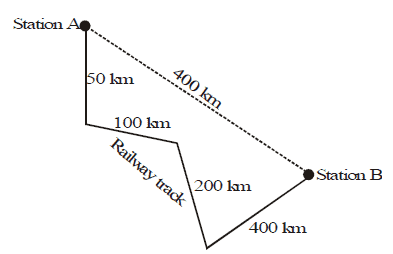Sol. (i)    Distance travelled by the train = 50 + 100 + 200 + 400 = 750 km.
(ii) Magnitude of the displacement in going from station A to station B = 400 km.

 DIFFERENCES BETWEEN DISTANCE AND DISPLACEMENT S.No Distance Displacement 1 It is defined as the actual path traversed by a body. It is the shortest distance between two points which the body moves. 2 It is a scalar quantity It is a vector quantity 3 It can never be negative or zero It can be negative, zero or positive. 4 Distance can be equal to or greater than displacement Displacement can be equal to or less than distance. 5 Distance travelled is not a unique path between two points. Displacement is a unique path between two points. 6 The distance between two points gives full information of the type of path followed by the body. Displacement between two points does not give full information of the type of path followed by the body. 7 Distance never decreases with time. for a moving body it is never zero. Displacement can decrease with time for a moving body it can be zero. 8 Distance in SI is measured in metre Displacement in SI is measured in metre.

UNIFORM AND NON-UNIFORM MOTION
A moving body may cover equal distances in equal intervals of time or different distances in equal intervals of time. On the basis of above assumption, the motion of a body can be classified as uniform motion and non-uniform motion.

Uniform motion: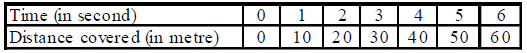When a body covers equal distances in equal intervals of time however small may be time intervals, the body is said to describe a uniform motion.
Example of uniform motion –

(i) An aeroplane flying at a speed of 600 km/h
(ii) A train running at a speed of 120 km/h
(iii) Light energy travelling at a speed of 3 × 108 m/s

Non-uniform motion: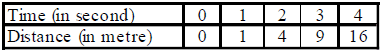When a body covers unequal distances in equal intervals of time, the body is said to be moving with a nonuniform motion.

Example of non-uniform motion –
(i) An aeroplane running on a runway before taking off.
(ii) A freely falling stone under the action of gravity.
(iii) An object thrown vertically upward.
(iv) When the brakes are applied to a moving car.

Velocity H The velocity of a body is the displacement of a body per unit time.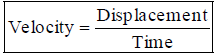The displacement covered by a body per unit time or the speed of a body in specified direction is called the velocity.

Unit: In SI system: m/s or ms-1

In CGS system: cm/s or cms-1

Other km/h or kmh-1, km/min.

Uniform velocity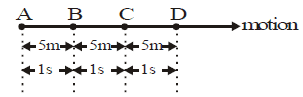Body moving with uniform velocity

When a body covers equal displacement in equal intervals of time, the body is said to be moving with a uniform velocity.

Conditions for uniform velocity:-

(i) The body must cover equal displacement in equal intervals of time.

(ii) The direction of motion of the body should not change.

Ex. (i) A train running towards south with a speed of 120 km/h.

(ii) An aeroplane flying due north-east with a speed of 600 km/h.

Very important note :-

The direction of velocity represents the direction of motion of body.

OR

Sign of velocity represents the direction of motion of body.

Non-uniform velocity/variable velocity :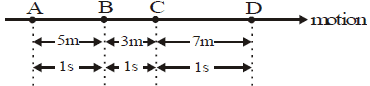Body moving with non-uniform velocity

When a body covers unequal displacement in equal intervals of time, the body is said to be moving with variable velocity.Body moving with variable velocity

When a body covers equal distances in equal intervals of time, but its direction changes, then the body is said to be moving with variable velocity.

Conditions for variable velocity:

(i) It should cover unequal displacement in equal intervals of time.

(ii) It should cover equal distances in equal intervals of time but its direction must change.

Ex. (i) A car running towards north on a busy road has a variable velocity as the displacement covered by it per unit time changes with change in the road condition.

(ii) The blades of a rotating ceiling fan, a person running around a circular track with constant speed etc. are the example of variable velocity, as the direction of the moving body changes in each case.

Average velocity:

Total displacement divided by total time is called average velocity.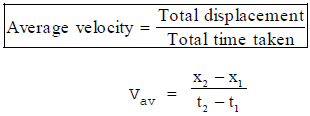OR

The arithmetic mean of initial velocity and final velocity for a given time period, is called average velocity.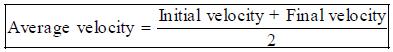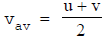where u = initial velocity, v = final velocity

Memorize: When a body moves with constant velocity, the average velocity is equal to instantaneous velocity. The body is said to be in uniform motion.

Difference between Speed and Velocity

 Speed Velocity It is defined as the rate of change of distance It is the shortest distance between two points between which the body moves. It is a scalar quantity It is a vector quantity It can never be negative or zero It can be negative, zero or positive Speed is velocity without direction. Velocity is directed speed Speed may or may not be equal to velocity. A body mass possesses different velocities but at the same speed. Speed never decreases with time. For a moving body it is never zero. Velocity can decrease with time. For a moving body it can be zero. Speed in SI is measured in ms–1 Velocity in SI, is measured in ms–1.

Acceleration

The rate of change of velocity of a moving body with time is called acceleration.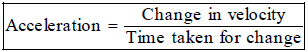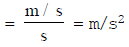but change in velocity = final velocity - initial velocity.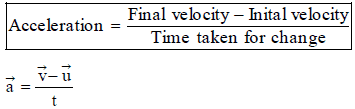If body moves with uniform velocity, then v = u and then acceleration is zero i.e. a = o.

Unit of acceleration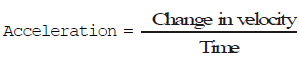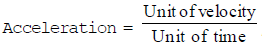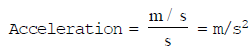In SI system is m/s2 or ms-2

In CGS system is cm/s2 or cms-2

Positive Acceleration: If the velocity of an object increases with time in the direction of the motion of the object, then the acceleration of the body is known as positive acceleration.

In this case, the object picks up the speed in a particular direction (i.e., velocity). For example, if an object starts from rest and its velocity goes on increasing with time in the direction of its motion, then the object has positive acceleration. The direction of positive acceleration is in the direction of motion of the object.

Negative Acceleration: If the velocity of an object decreases with time, then the acceleration of the object is known as negative acceleration.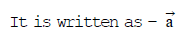For example, if an object moving with certain velocity is brought to rest then the object is said to have negative acceleration.

Acceleration without changing speed:

When an object moves in a circular path with constant speed, then its velocity changes due to the change in the direction of motion of the object. hence, the object is accelerated without changing its speed.

In this case, the direction of acceleration is towards the centre of the circular path.

• Positive or negative sign of acceleration always shows the direction of acceleration or direction of force but not represent direction of motion of body.
• Acceleration which opposes the motion of a body is called retardation or negative acceleration.
• If sign of velocity and acceleration are same it means speed of body will always increase.
• If both are opposite sign it means speed of body will always decrease.

Uniform acceleration: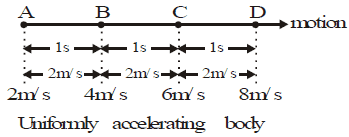When a body undergoes equal changes in velocity in equal intervals of time, the body is said to be moving with a uniform acceleration

Ex. (i) Motion of a freely falling body.

(ii) Motion of a ball rolling down an inclined plane.

Non-uniform acceleration or variable acceleration :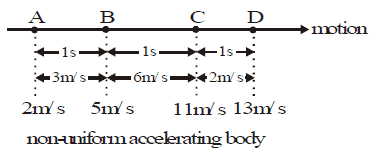When a body describes unequal change in velocity in equal intervals of time, the body is said to be moving with non-uniform acceleration.

Ex. (i) The motion of a bus leaving or entering the bus stop.

(ii) The motion of a train leaving or entering the platform.

(iii) A car moving on a busy road has non-uniform acceleration.

Offer running on EduRev: Apply code STAYHOME200 to get INR 200 off on our premium plan EduRev Infinity!

,

,

,

,

,

,

,

,

,

,

,

,

,

,

,

,

,

,

,

,

,

;